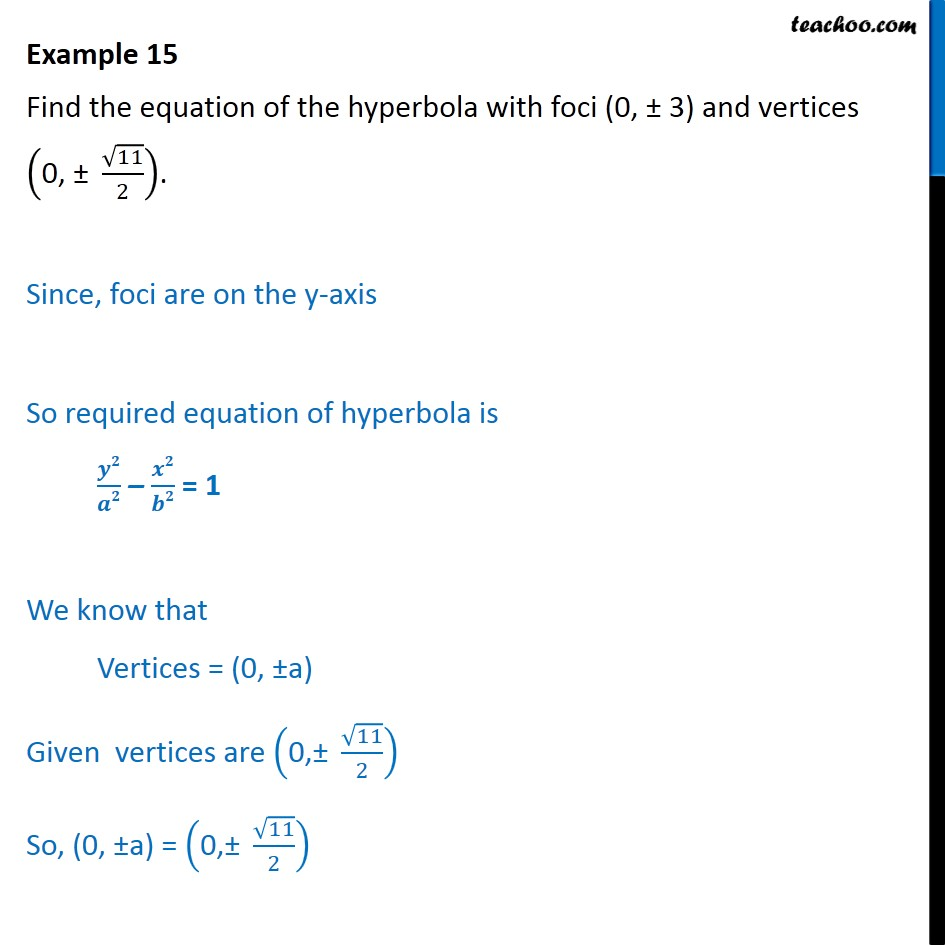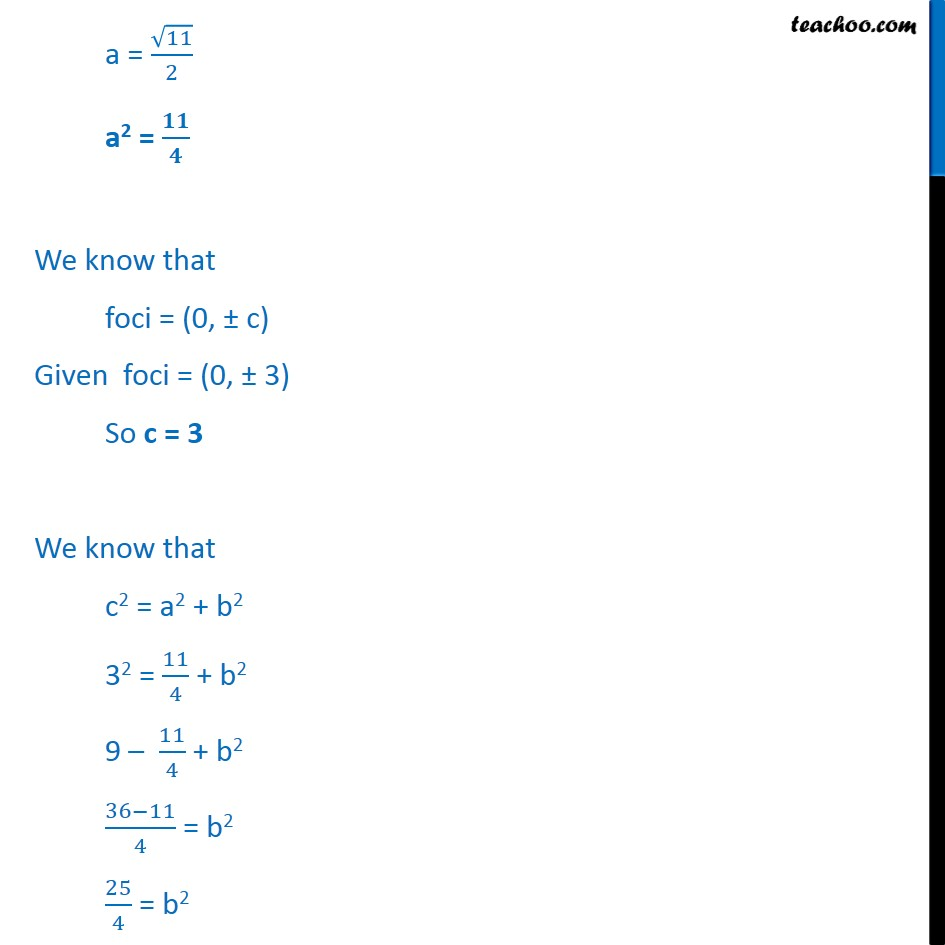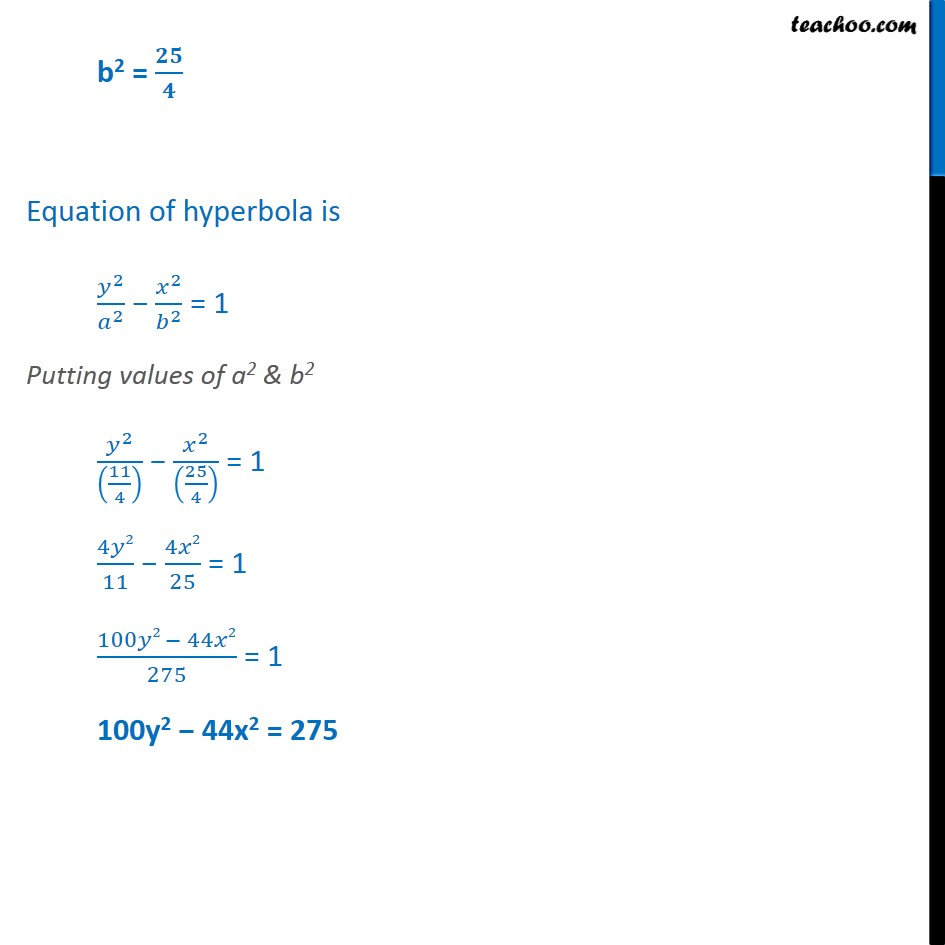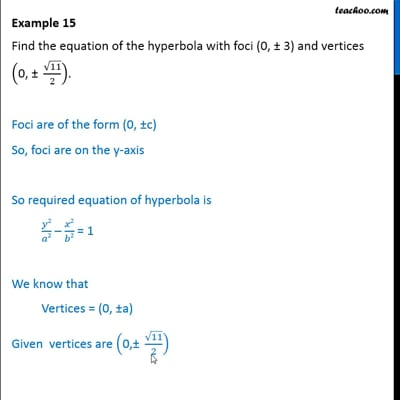Examples

Chapter 10 Class 11 Conic Sections
Serial order wiseThis video is only available for Teachoo black users

Learn in your speed, with individual attention - Teachoo Maths 1-on-1 Class

### Transcript

Example 15 Find the equation of the hyperbola with foci (0, 3) and vertices 0, 11 2 . Since, foci are on the y-axis So required equation of hyperbola is = 1 We know that Vertices = (0, a) Given vertices are 0, 11 2 So, (0, a) = 0, 11 2 a = 11 2 a2 = We know that foci = (0, c) Given foci = (0, 3) So c = 3 We know that c2 = a2 + b2 32 = 11 4 + b2 9 11 4 + b2 36 11 4 = b2 25 4 = b2 b2 = Equation of hyperbola is 2 2 2 2 = 1 Putting values of a2 & b2 2 11 4 2 25 4 = 1 4 2 11 4 2 25 = 1 100 2 44 2 275 = 1 100y2 44x2 = 275